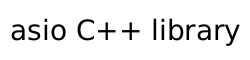#### basic_streambuf::size

Get the size of the input sequence.

```std::size_t size() const;
```
###### Return Value

The size of the input sequence. The value is equal to that calculated for `s` in the following code:

```size_t s = 0;
const_buffers_type bufs = data();
const_buffers_type::const_iterator i = bufs.begin();
while (i != bufs.end())
{
const_buffer buf(*i++);
s += buf.size();
}
```